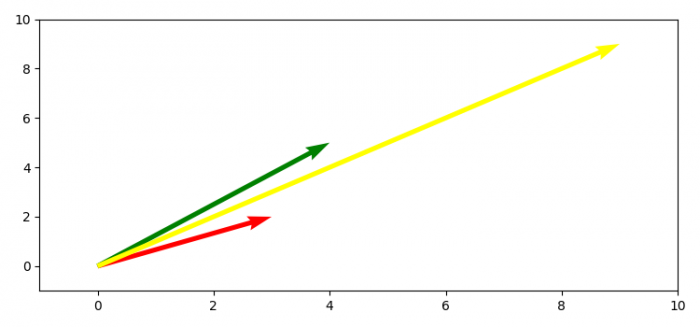# How to plot 2D math vectors with Matplotlib?

To plot 2D math vectors with matplotlib, we can take the following steps−

• Create vector cordinates using numpy array.
• Get x, y, u and v data points.
• Create a new figure or activate an existing figure using figure method.
• Get the current axis using gca() method.
• Set x an y limits of the axes.
• To redraw the current figure, use draw() method.
• To display the figure, use show() method.

## Example

import numpy as np
import matplotlib.pyplot as plt
plt.rcParams["figure.figsize"] = [7.50, 3.50]
plt.rcParams["figure.autolayout"] = True
soa = np.array([[0, 0, 3, 2], [0, 0, 4, 5], [0, 0, 9, 9]])
X, Y, U, V = zip(*soa)
plt.figure()
ax = plt.gca()
ax.quiver(X, Y, U, V, angles='xy', scale_units='xy', scale=1, color=['red', 'green', 'yellow'])
ax.set_xlim([-1, 10])
ax.set_ylim([-1, 10])
plt.draw()
plt.show()

## Output